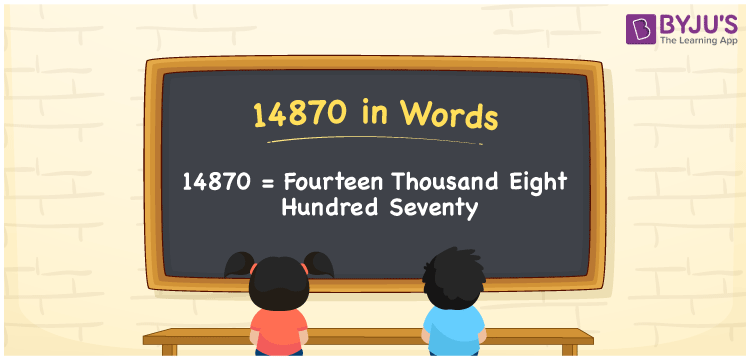# 14870 in Words

14870 in words is written as Fourteen Thousand Eight Hundred and Seventy. In 14870, 1 has a place value of ten thousand, 4 is in the place value of thousand, 8 is in the place value of hundred and 7 is in the place value of ten. The article on Place Value gives more information. The number 14870 is used in expressions that relate to money, distance, social media views, and many more. For example, “The Employee number of my colleague is Fourteen Thousand Eight Hundred and Seventy.”

 14870 in words Fourteen Thousand Eight Hundred and Seventy Fourteen Thousand Eight Hundred and Seventy in Numbers 14870

## 14870 in English Words## How to Write 14870 in Words?

We can convert 14870 in words using a place value chart. The number 14870 has 5 digits, so let’s make a chart that shows the place value up to 5 digits.

 Ten Thousand Thousands Hundreds Tens Ones 1 4 8 7 0

Thus, we can write the expanded form as:

1 × Ten thousand + 4 × Thousand + 8 × Hundred + 7 × Ten + 0 × One

= 1 × 10000 + 4 × 1000 + 8 × 100 + 7 × 10 + 0 × 1

= 14870.

= Fourteen Thousand Eight Hundred and Seventy.

14870 is the natural number that is succeeded by 14869 and preceded by 14871.

14870 in words – Fourteen Thousand Eight Hundred and Seventy.

Is 14870 an odd number? – No.

Is 14870 an even number? – Yes.

Is 14870 a perfect square number? – No.

Is 14870 a perfect cube number? – No.

Is 14870 a prime number? – No.

Is 14870 a composite number? – Yes.

## Solved Example

Question: Write the number 14870 in expanded form.

Solution: 1 x 10000 + 4 x 1000 + 8 x 100 + 7 x 10 + 0 x 1

We can write 14870 = 10000 + 4000 + 800 + 70 + 0

= 1 x 10000 + 4 x 1000 + 8 x 100 + 7 x 10 + 0 x 1.

## Frequently Asked Questions on 14870 in words

Q1

### How to write the number 14870 in words?

14870 in words is written as Fourteen Thousand Eight Hundred and Seventy.
Q2

### State whether True or False. 14870 is divisible by 2?

True. 14870 is divisible by 2.
Q3

### Is 14870 divisible by 10?

True. 14870 is divisible by 10.
Q4

### Is 14,870 a composite number?

Yes, 14,870 is a composite number as 14,870 has more than two factors.
Q5

### Is 14,870 an odd number?

No, 14,870 is an even number.